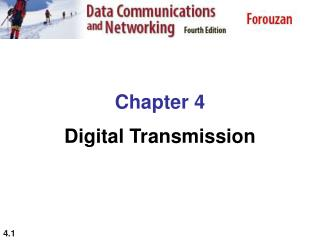DownloadDownload PresentationChapter 4 Digital Transmission

# Chapter 4 Digital Transmission

Download Presentation## Chapter 4 Digital Transmission

- - - - - - - - - - - - - - - - - - - - - - - - - - - E N D - - - - - - - - - - - - - - - - - - - - - - - - - - -
##### Presentation Transcript

1. Chapter 4 Digital Transmission

2. 4-1 DIGITAL-TO-DIGITAL CONVERSION In this section, we see how we can represent digital data by using digital signals. The conversion involves three techniques: line coding, block coding, and scrambling. Line coding is always needed; block coding and scrambling may or may not be needed. Topics discussed in this section: Line Coding Line Coding SchemesBlock Coding Scrambling

3. Figure 4.1 Line coding and decoding

4. Figure 4.2 Signal element versus data element

5. Example 4.1 A signal is carrying data in which one data element is encoded as one signal element ( r = 1). If the bit rate is 100 kbps, what is the average value of the baud rate if c is between 0 and 1? Solution We assume that the average value of c is 1/2 . The baud rate is then

6. Note Although the actual bandwidth of a digital signal is infinite, the effective bandwidth is finite.

7. Example 4.2 The maximum data rate of a channel (see Chapter 3) is Nmax = 2 × B × log2 L (defined by the Nyquist formula). Does this agree with the previous formula for Nmax? Solution A signal with L levels actually can carry log2L bits per level. If each level corresponds to one signal element and we assume the average case (c = 1/2), then we have

8. Figure 4.3 Effect of lack of synchronization

9. Example 4.3 In a digital transmission, the receiver clock is 0.1 percent faster than the sender clock. How many extra bits per second does the receiver receive if the data rate is 1 kbps? How many if the data rate is 1 Mbps? Solution At 1 kbps, the receiver receives 1001 bps instead of 1000 bps. At 1 Mbps, the receiver receives 1,001,000 bps instead of 1,000,000 bps.

10. Figure 4.4 Line coding schemes

11. Figure 4.5 Unipolar NRZ scheme

12. Figure 4.6 Polar NRZ-L and NRZ-I schemes

13. Note In NRZ-L the level of the voltage determines the value of the bit. In NRZ-I the inversion or the lack of inversion determines the value of the bit.

14. Note NRZ-L and NRZ-I both have an average signal rate of N/2 Bd.

15. Note NRZ-L and NRZ-I both have a DC component problem.

16. Example 4.4 A system is using NRZ-I to transfer 10-Mbps data. What are the average signal rate and minimum bandwidth? Solution The average signal rate is S = N/2 = 500 kbaud. The minimum bandwidth for this average baud rate is Bmin = S = 500 kHz.

17. Figure 4.7 Polar RZ scheme

18. Figure 4.8 Polar biphase: Manchester and differential Manchester schemes

19. Note In Manchester and differential Manchester encoding, the transition at the middle of the bit is used for synchronization.

20. Note The minimum bandwidth of Manchester and differential Manchester is 2 times that of NRZ.

21. Note In bipolar encoding, we use three levels: positive, zero, and negative.

22. Figure 4.9 Bipolar schemes: AMI and pseudoternary

23. Note In mBnL schemes, a pattern of m data elements is encoded as a pattern of n signal elements in which 2m ≤ Ln.

24. Figure 4.10 Multilevel: 2B1Q scheme

25. Figure 4.11 Multilevel: 8B6T scheme

26. Figure 4.12 Multilevel: 4D-PAM5 scheme

27. Figure 4.13 Multitransition: MLT-3 scheme

28. Table 4.1 Summary of line coding schemes

29. Note Block coding is normally referred to as mB/nB coding; it replaces each m-bit group with an n-bit group.

30. Figure 4.14 Block coding concept

31. Table 4.2 4B/5B mapping codes

32. Figure 4.16 Substitution in 4B/5B block coding

33. Example 4.5 We need to send data at a 1-Mbps rate. What is the minimum required bandwidth, using a combination of 4B/5B and NRZ-I or Manchester coding? Solution First 4B/5B block coding increases the bit rate to 1.25 Mbps. The minimum bandwidth using NRZ-I is N/2 or 625 kHz. The Manchester scheme needs a minimum bandwidth of 1 MHz. The first choice needs a lower bandwidth, but has a DC component problem; the second choice needs a higher bandwidth, but does not have a DC component problem.

34. Figure 4.17 8B/10B block encoding

35. Figure 4.18 AMI used with scrambling

36. Figure 4.19 Two cases of B8ZS scrambling technique

37. Note B8ZS substitutes eight consecutive zeros with 000VB0VB.

38. Note HDB3 substitutes four consecutive zeros with 000V or B00V depending on the number of nonzero pulses after the last substitution.

39. Figure 4.29 Delta modulation components

40. Figure 4.30 Delta demodulation components